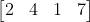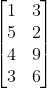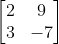1-646-564-2231

# How to Multiply Matrices? With ExamplesMatrix multiplication is one of the fundamental yet advanced concepts of matrices. You have to be careful while you multiply matrices. It is not as easy as it sounds. You should have sound knowledge of all the basic concepts like what a matrix is, the rows and columns in a matrix, how to represent a matrix, and how to multiply matrices.

Back to school: Matrices are the groupings of numbers, variables, symbols, or expressions in a rectangular table with varied numbers of rows and columns. They are rectangular arrays with different operations like addition, multiplication, and transposition. The elements of a matrix are the numbers or entries that make up the matrix. The horizontal elements of matrices are rows, whereas the vertical elements are columns.

You can depict the number of rows and columns with the help of variables. For example, let the number of rows in matrix K be ‘m’ and the number of columns be ‘n’. Thus we can represent the matrix K as [K] m x n. Matrix is represented inside the square brackets ‘[ ]’ with the product of its rows and columns in the subscript.

Until 1812 matrix multiplication was not known to mankind. Philippe Marie Binet, a French mathematician, invented matrix multiplication in 1812 to describe linear maps with matrices. He discovered that matrix multiplication is a binary operation. Two matrices multiply to form a single matrix.

Further in this article, you shall learn the rules and major concepts for multiplying matrices.

## What is Matrix Multiplication?

In the introduction of this article, you read that matrix multiplication is a binary operation. This means that whenever you find the product of two or more matrices, the answer is a single matrix, which follows the initial matrices. For multiplying two matrices, their compatibility is checked. This means that two matrices must follow a set of rules to be multiplied.

These compatibility parameters are described below:

• The value of the number of columns in matrix one should be equal to the value of the number of rows in matrix 2.
• If the above condition doesn’t satisfy, the matrices cannot be multiplied.
• If the matrices are square matrices with the same order, they can be multiplied by each other.
•  A square matrix of 2 x 2 cannot be multiplied with a square matrix of 3 x 3. It can be multiplied with another square matrix of 2 x 2.

Let us take an example to understand this in a better way:

Example 1: Suppose we have two matrices, K and L. The order of the matrices is given as m x n and n x o, respectively. Find whether the matrices can be multiplied or not?

Solution: We are given

Matrix 1 = [ K ] m x n

Matrix 2 = [ L ] n x o

We can see that the number of columns in matrix K equals the number of rows in matrix L. Hence the two matrices can be multiplied.

Resultant Matrix: The matrix achieved by multiplying two matrices is the resultant matrix. This matrix can be represented as:

• The name of the matrix in square brackets
• The product of the number of rows of matrix 1 with the number of columns of matrix two as the order.

Again let us look at the example mentioned above:

We know that K and L are compatible with multiplication. So the resultant matrix, say D, would be = [ D ] m x o where m = number of rows of matrix one and o = the number of columns of matrix 2.

Example 2: A matrix [ K ] 2 x 4 is multiplied by the matrix [ X ] 4 x 2. If [ Y ] is the resultant matrix, then what is the order of Y.

Solution: We are given

Matrix 1 = [ K ] 2 x 4

Matrix 2 = [ X ] 4 x 2

Since the number of columns of matrix K equals the number of rows of matrix X, K and X can be multiplied. Therefore,

Matrix Y = [ Y ] 2 x 2

The order of matrix Y is 2 x 2. Y is a square matrix.

The orientation of 2 matrices is also a crucial factor for determining the product of the matrices. If there are two matrices, K and L, then to find the product of K and L, you must first write the matrix K and then write matrix L. If you write the matrix L before matrix K, you will get a completely different matrix. Commutative law doesn’t apply during matrix multiplication. This means KL ≠ LK.

Now that we are clear with the rules for the multiplication of two matrices, let us learn how to multiply matrices.

## How to Multiply Matrices?

Let us learn the stepwise procedure for multiplying two matrices. At first, you may find it confusing but when you get the hang of it, multiplying matrices is as easy as applying butter to your toast.

Step 1: Check the compatibility of the matrices given. If they are not compatible, leave the multiplication.

Step 2: Take the first row of matrix 1 and multiply it with the first column of matrix 2. Then multiply the first row of matrix 1 with the 2nd column of matrix 2. Now multiply the first row of matrix 1 with the 3rd column of matrix 2, and so on. The values obtained from these will fill the first row of the product matrix.

Step 3: Now take the second row of matrix 1 and multiply it with the first column of matrix 2 and follow the same steps as 2. This will fill the second row of the product matrix.

Step 4: Continue these steps by taking each row until the product matrix is obtained.

By multiplying rows and columns we mean that the elements present in those rows and columns will be multiplied. Remember to multiply the corresponding elements and then add the products to find the element of the product matrix.

If you aren’t sure about the steps then look at the step-by-step solved example below:

Example: Find the product of the matrices given below:

K =and L =Solution:

Step 1: The matrix K is of the order 1 x 4 and the matrix L is of the order 4 x 2. Since the number of columns of matrix K matches the number of rows of matrix L, therefore the matrices are compatible for multiplication. The resultant matrix is of the order 1 x 2.

Step 2: Since K has only 1 row, multiply it with the first column of matrix L in this way: (2 x 4) + (4 x 5) + (1 x 4) + (7 x 3) = 51. Note how the 1st element of the row is multiplied with the 1st element of column 1, similarly to the 2nd, 3rd, and 4th. They are then added together to get the resultant matrix element.

Step 3: Likewise now multiply the 1st row with the second column of matrix L. The result will be (2 x 3) + (4 x 2) + (1 x 9) + (7 x 6) = 65.

Step 4: Arrange the result in the product matrix. Let’s suppose [ X ] is the resultant matrix; therefore, X = [51   65].

We just learned how to multiply two matrices. The next section will learn how to solve a ( 2 x 2 ) square matrix.

We just learned how to multiply two matrices. The next section will learn how to solve a ( 2 x 2 ) square matrix.

## How to Multiply 2 x 2 Matrix

For multiplying matrices 2 x 2, you should be well versed with the steps mentioned in the above section. Since we are multiplying 2 square matrices of the same order, we don’t need to check the compatibility in this case. Remember that the product matrix will also be in the same order as the square matrix. Let us solve an example to understand how to multiply the matrix of 2 x 2.

Example: Multiply the square matrix

A =and B =Step 1: Multiplying the first row of matrix A with the first column of matrix B. We get (2 x 1) + (9 x 3) = 29. Now multiplying the first row of matrix A with the second column of matrix B. We get (2 x -4) + (9 x 7) = -8 + 63 = 55.

Step 2: We will repeat step 1 but change the row of matrix A. The first and second columns of matrix B will be multiplied by row 2 of matrix A. The results are

(3 x 1) + (-7 x 3) = 3 – 21 = -18, and (3 x -4) + (-7 x 7) = -12 – 49 = -61

Step 3: Place the results properly

.

Therefore the resultant matrix, C =Similarly, you can solve square matrices like 3 x 3, 4 x 4, and so on.

### TESTIMONIALS## More Related TOPICS

### Use Models to Subtract Mixed Numbers

Key Concepts Using models to subtract mixed numbers Subtract mixed numbers Addition and subtraction of mixed

### Use Models to Add Mixed Numbers

Key Concepts Using models to add mixed numbers Adding mixed numbers Introduction  In this chapter, we

### Find Common Denominators

Key Concepts Equivalent fractions and common denominators Finding common denominators Introduction In this chapter, we will